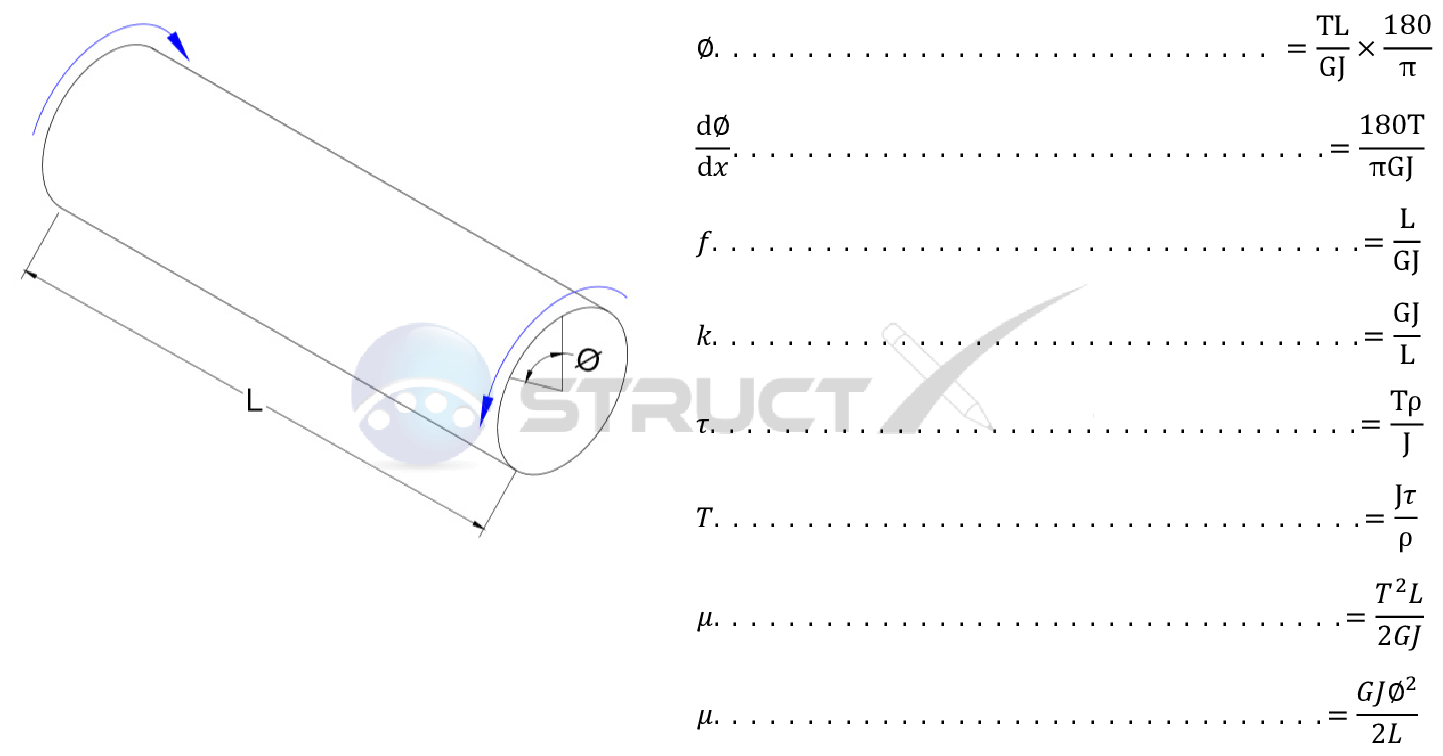# Notation and Units

## Metric and Imperial Units

The above formulas may be used with both imperial and metric units. As with all calculations care must be taken to keep consistent units throughout with examples of units which should be adopted listed below:

# Notation

• L = length under consideration, in or mm
• f = torsional flexibility, psi or MPa
• G = shear modulus or modulus of rigidity, psi or MPa
• J = polar moment of inertia, in4 or mm4
• k = torsional stiffness, lb.in2 or Nmm2
• T = applied or resulting torsion, lb.in or Nmm
• θ = angle of twist, degrees
• τ = shear stress, psi or MPa
• ρ = radial distance from centre, in or mm
• µ = strain energy, ib.in or Nmm
• dθ/dx = rate of twist, degrees/unit